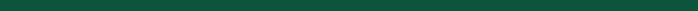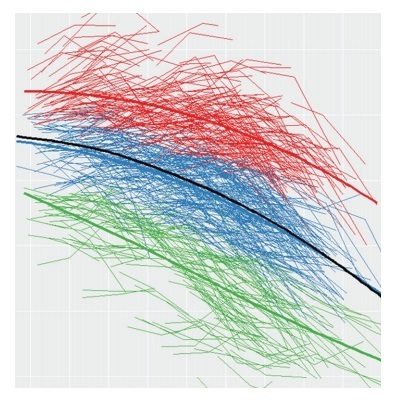Like   Tweet   in

## Announcing JAD, Volume 70, Supplement 1, 2019

 /* styles */
 table.module-4{width:99.62%;padding:0}table div table+table+table+table+table div table{width:99.62%;float:none;margin-left:auto;margin-right:auto;padding:0}table div table+table+table+table+table div table a{border:0 none;text-decoration:none}table div table+table+table+table+table div table img{width:100%!important;border:0 none;text-decoration:none}table div table+table+table+table+table div table td{width:100%;padding:0}/* styles */# Dementia, Risk, Risk Reduction, and Translation into Practice

## International Research Network for Dementia Prevention Special Issue

 table div table+table+table+table+table+table+table+table+table div table,table.module-8{width:25.28%;float:right;padding:0}table div table+table+table+table+table+table+table+table+table div table a{border:0 none;text-decoration:none}table div table+table+table+table+table+table+table+table+table div table img{width:100%!important;border:0 none;text-decoration:none}table div table+table+table+table+table+table+table+table+table div table td{width:100%;padding:0 0 20px 20px}/* styles */ We are pleased to announce a new special issue of the Journal of Alzheimer's Disease (JAD) (Volume 70, Supplmement 1) is available now. In this open access issue from the International Research Network on Dementia Prevention (IRNDP) new research from around the globe examining the potential risk factors for dementia is presented. The special issue has been compiled by guest editors Kaarin Anstey and Ruth Peters at Neuroscience Research Australia (NeuRA) and the University of New South Wales (UNSW). "We report on some of the ongoing and crucial risk reduction trials and highlight some of the population level considerations and the remaining evidence gaps," comment the guest editors. "The content demonstrates impressive progress in dementia prevention research and provides an invaluable collation of recent research and an up to date overview of the dementia prevention evidence base. Yet it also serves to highlight the substantial remaining evidence gaps and the next steps forward in this area of research."## Guest Editors# Prof. Kaarin Anstey

Kaarin Anstey, PhD, is a Senior Principal Research Scientist at NeuRA and leads an innovative multidisciplinary team addressing ageing research. Her research focuses on the causes, consequences and prevention of cognitive ageing, dementia, and common mental disorders in adulthood. Kaarin leads an NHMRC Centre of Research Excellence in Cognitive Health, is a director of the NHMRC Dementia Centre for Research Collaboration and Co-Deputy Director of the ARC Centre of Excellence in Population Ageing Research, and is a Scientia Professor at UNSW.# Dr. Ruth Peters

Ruth Peters, PhD, is a Senior Research Scientist at NeuRA and works to develop our understanding of the risk factors which increase our risk of cognitive decline and dementia and the ways in which we might act to reduce this risk. Alongside leading teams delivering high profile evidence synthesis work in the area of dementia risk reduction, Ruth has a particular interest in hypertension and in the treatment of hypertension in older adults, and she has a conjoint position as Senior Lecturer at UNSW.

## Click here to view the related blog post by the guest editors on J-Alz.com!## Dementia, Risk, Risk Reduction, and Translation into Practice

Anstey, K | Peters, R (Editorial in JAD 70:s1)

 table div table+table+table+table+table+table+table+table+table+table+table+table+table+table+table+table+table+table div table{width:100%;padding:0}table div table+table+table+table+table+table+table+table+table+table+table+table+table+table+table+table+table+table div table img{width:96.23%;padding:0;float:none}table div table+table+table+table+table+table+table+table+table+table+table+table+table+table+table+table+table+table div table td{width:100%;padding:0 1.88% 18px}/* styles */## Using Cross-Cultural Studies to Improve Evidence on Dementia Prevention

Glymour, M. Maria | Whitmer, Rachel A. (Editorial in JAD 70:s1)

 table div table+table+table+table+table+table+table+table+table+table+table+table+table+table+table+table+table+table+table+table div table{width:100%;padding:0}table div table+table+table+table+table+table+table+table+table+table+table+table+table+table+table+table+table+table+table+table div table img{width:96.23%;padding:0;float:none}table div table+table+table+table+table+table+table+table+table+table+table+table+table+table+table+table+table+table+table+table div table td{width:100%;padding:0 1.88% 18px}/* styles */## Prevention of Cognitive Decline: A Goal in Sight?

Brayne, Carol | Richard, Edo (Editorial in JAD 70:s1)

 table div table+table+table+table+table+table+table+table+table+table+table+table+table+table+table+table+table+table+table+table+table+table div table{width:100%;padding:0}table div table+table+table+table+table+table+table+table+table+table+table+table+table+table+table+table+table+table+table+table+table+table div table img{width:96.23%;padding:0;float:none}table div table+table+table+table+table+table+table+table+table+table+table+table+table+table+table+table+table+table+table+table+table+table div table td{width:100%;padding:0 1.88% 18px}/* styles */## Population-Based Approaches to Dementia Prevention

Sexton, Claire E. | Yaffe, Kristine (Editorial in JAD 70:s1)# JAD, Volume 70, Supplement 1 / 2019 Available Online

This open access special issue includes one review article and 18 research articles. A selection of content is featured below; click on the titles to access the articles. To view the entire issue, go here.

 table div table+table+table+table+table+table+table+table+table+table+table+table+table+table+table+table+table+table+table+table+table+table+table+table+table+table+table div table{width:100%;padding:0}table div table+table+table+table+table+table+table+table+table+table+table+table+table+table+table+table+table+table+table+table+table+table+table+table+table+table+table div table img{width:96.23%;padding:0;float:none}table div table+table+table+table+table+table+table+table+table+table+table+table+table+table+table+table+table+table+table+table+table+table+table+table+table+table+table div table td{width:100%;padding:0 1.88% 18px}/* styles */## Modifiable Risk Factors Discriminate Memory Trajectories in Non-Demented Aging: Precision Factors and Targets for Promoting Healthier Brain Aging and Preventing Dementia

McFall, G. Peggy | McDermott, Kirstie L. | Dixon, Roger A.Non-demented cognitive aging trajectories are characterized by vast level and slope differences and a spectrum of outcomes, including dementia.This study has two main objectives: first, longitudinal data of memory trajectories to operationally define separable clusters; and, second, a machine-learning computational technology to test 17 risk predictors of memory status class. The predictors derive from four AD risk domains: non-modifiable demographic, functional biomarker, psychological, and lifestyle. Given both age- and sex-related differences in AD incidence, age and sex may be selective predictors.

 table div table+table+table+table+table+table+table+table+table+table+table+table+table+table+table+table+table+table+table+table+table+table+table+table+table+table+table+table+table+table div table{width:100%;padding:0}table div table+table+table+table+table+table+table+table+table+table+table+table+table+table+table+table+table+table+table+table+table+table+table+table+table+table+table+table+table+table div table img{width:96.23%;padding:0;float:none}table div table+table+table+table+table+table+table+table+table+table+table+table+table+table+table+table+table+table+table+table+table+table+table+table+table+table+table+table+table+table div table td{width:100%;padding:0 1.88% 18px}/* styles */## Impacts of Overweight and Obesity in Older Age on the Risk of Dementia: A Systematic Literature Review and a Meta-Analysis

Danat, Isaac M. | Clifford, Angela | Partridge, Martin | Zhou, Weiju | Bakre, Aishat T. | Chen, Anthony | McFeeters, Danielle | Smith, Tina | Wan, Yuhui | Copeland, John | Anstey, Kaarin J. | Chen, Ruoling

 /* styles */ It is unclear whether overweight and obesity in older age reduces or increases the risk of incident dementia. In this paper, we carried out a comprehensive systematic literature review and meta-analysis to assess the impact of obesity and overweight measured by body mass index (BMI) and other indicators of central fat in older age on incident dementia. We added new data from an unpublished study in China to examine whether the length of the cohort follow-up affected the findings of the association between overweight/obesity in older age and incident dementia.
 table div table+table+table+table+table+table+table+table+table+table+table+table+table+table+table+table+table+table+table+table+table+table+table+table+table+table+table+table+table+table+table+table+table div table{width:100%;padding:0}table div table+table+table+table+table+table+table+table+table+table+table+table+table+table+table+table+table+table+table+table+table+table+table+table+table+table+table+table+table+table+table+table+table div table img{width:96.23%;padding:0;float:none}table div table+table+table+table+table+table+table+table+table+table+table+table+table+table+table+table+table+table+table+table+table+table+table+table+table+table+table+table+table+table+table+table+table div table td{width:100%;padding:0 1.88% 18px}/* styles */## Chronic Obstructive Pulmonary Disease and Risk of Dementia and Mortality in Lower to Middle Income Countries

Cherbuin, Nicolas | Walsh, Erin I. | Prina, A. Matthew

 /* styles */ The main focus of this study is around the epidemiology of dementia, although the scope of the research is much broader, including other health domains (chronic diseases, disability, frailty, poly-morbidity) and social aspects of aging. Leveraging against this rich resource, the aims of this study were to investigate: (1) the prevalence of chronic obstructive pulmonary disease (COPD); and (2) the prospective risk of dementia and mortality attributable to COPD in older community-living individuals living in eight lower to middle income countries.
 table div table+table+table+table+table+table+table+table+table+table+table+table+table+table+table+table+table+table+table+table+table+table+table+table+table+table+table+table+table+table+table+table+table+table+table+table div table{width:100%;padding:0}table div table+table+table+table+table+table+table+table+table+table+table+table+table+table+table+table+table+table+table+table+table+table+table+table+table+table+table+table+table+table+table+table+table+table+table+table div table img{width:96.23%;padding:0;float:none}table div table+table+table+table+table+table+table+table+table+table+table+table+table+table+table+table+table+table+table+table+table+table+table+table+table+table+table+table+table+table+table+table+table+table+table+table div table td{width:100%;padding:0 1.88% 18px}/* styles */## Social Isolation and Cognitive Function in Later Life: A Systematic Review and Meta-Analysis

Evans, Isobel E.M. | Martyr, Anthony | Collins, Rachel | Brayne, Carol | Clare, Linda

 /* styles */ Given that social isolation may be associated with poor cognitive function in later life, we aimed to investigate, through a systematic review and meta-analysis of data from longitudinal cohort studies, the relationship between aspects of social isolation (including social activity and social networks) and cognitive function in community-dwelling older people. We considered studies that assessed cognition using validated measures of global cognition, as these are frequently used, and measures of memory and executive function, as change in these domains is central to the concept of cognitive aging
 table div table+table+table+table+table+table+table+table+table+table+table+table+table+table+table+table+table+table+table+table+table+table+table+table+table+table+table+table+table+table+table+table+table+table+table+table+table+table+table div table{width:100%;padding:0}table div table+table+table+table+table+table+table+table+table+table+table+table+table+table+table+table+table+table+table+table+table+table+table+table+table+table+table+table+table+table+table+table+table+table+table+table+table+table+table div table img{width:96.23%;padding:0;float:none}table div table+table+table+table+table+table+table+table+table+table+table+table+table+table+table+table+table+table+table+table+table+table+table+table+table+table+table+table+table+table+table+table+table+table+table+table+table+table+table div table td{width:100%;padding:0 1.88% 18px}/* styles */table.module-40{width:27.36%;padding:0}table div table+table+table+table+table+table+table+table+table+table+table+table+table+table+table+table+table+table+table+table+table+table+table+table+table+table+table+table+table+table+table+table+table+table+table+table+table+table+table+table+table div table{width:27.36%;float:none;margin-left:auto;margin-right:auto;padding:0}table div table+table+table+table+table+table+table+table+table+table+table+table+table+table+table+table+table+table+table+table+table+table+table+table+table+table+table+table+table+table+table+table+table+table+table+table+table+table+table+table+table div table a{border:0 none;text-decoration:none}table div table+table+table+table+table+table+table+table+table+table+table+table+table+table+table+table+table+table+table+table+table+table+table+table+table+table+table+table+table+table+table+table+table+table+table+table+table+table+table+table+table div table img{width:100%!important;border:0 none;text-decoration:none}table div table+table+table+table+table+table+table+table+table+table+table+table+table+table+table+table+table+table+table+table+table+table+table+table+table+table+table+table+table+table+table+table+table+table+table+table+table+table+table+table+table div table td{width:100%;padding:0}/* styles */table div table+table+table+table+table+table+table+table+table+table+table+table+table+table+table+table+table+table+table+table+table+table+table+table+table+table+table+table+table+table+table+table+table+table+table+table+table+table+table+table+table+table+table+table div table,table.module-43{width:38.49%;float:right;padding:0}table div table+table+table+table+table+table+table+table+table+table+table+table+table+table+table+table+table+table+table+table+table+table+table+table+table+table+table+table+table+table+table+table+table+table+table+table+table+table+table+table+table+table+table+table div table a{border:0 none;text-decoration:none}table div table+table+table+table+table+table+table+table+table+table+table+table+table+table+table+table+table+table+table+table+table+table+table+table+table+table+table+table+table+table+table+table+table+table+table+table+table+table+table+table+table+table+table+table div table img{width:100%!important;border:0 none;text-decoration:none}table div table+table+table+table+table+table+table+table+table+table+table+table+table+table+table+table+table+table+table+table+table+table+table+table+table+table+table+table+table+table+table+table+table+table+table+table+table+table+table+table+table+table+table+table div table td{width:100%;padding:0 0 20px 20px}/* styles */ The International Research Network on Dementia Prevention (IRNDP) is a multinational network working to increase dementia risk reduction messaging worldwide, and develop targeted research to answer questions about the detailed impact of known and emerging risk factors in dementia. Based in Australia, IRNDP aims to link researchers globally to foster new research and accelerate knowledge translation that will delay or prevent dementia worldwide. The IRNDP Conference 2019 will be held in Sydney, Australia on October 14 and 15. The event is free to attend and the registration deadline is October 1. Full details are available at the event website.## Help Spread the News of this Special Issue!table div table+table+table+table+table+table+table+table+table+table+table+table+table+table+table+table+table+table+table+table+table+table+table+table+table+table+table+table+table+table+table+table+table+table+table+table+table+table+table+table+table+table+table+table+table+table+table+table+table div table{width:100%;padding:0}table div table+table+table+table+table+table+table+table+table+table+table+table+table+table+table+table+table+table+table+table+table+table+table+table+table+table+table+table+table+table+table+table+table+table+table+table+table+table+table+table+table+table+table+table+table+table+table+table+table div table img{width:96.23%;padding:0;float:none}table div table+table+table+table+table+table+table+table+table+table+table+table+table+table+table+table+table+table+table+table+table+table+table+table+table+table+table+table+table+table+table+table+table+table+table+table+table+table+table+table+table+table+table+table+table+table+table+table+table div table td{width:100%;padding:0 1.88% 18px}/* styles */